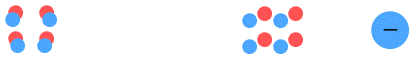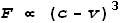Home

# Static Electricity

Static electricity refers to an electrical charge that builds up due to contact between electrically dissimilar materials. Literally “electricity at rest”, it is understood to be caused by electrons being transferred from an object that attracts them weakly to an object that attracts them strongly. The extent to which an object attracts electrons is referred to as its ‘dielectric’ properties.

When two objects with different dielectrics come into contact the result is that one of the objects gains a net negative charge and the other a net positive charge. Once charged, the objects then display some unusual behaviour:

1. Objects with a net negative or net positive charge can attract other objects having a net neutral charge. This appears to violate Coulomb’s Law which states that only objects with opposite charge should attract. In other words, we should expect no attraction to occur.

2. Objects with a strong negative charge can attract objects with a weak negative charge. This appears to fully violate Coulomb’s Law which predicts that such objects should repel. If the like-charges are of a similar strength then they do indeed repel, as would be expected. But attraction appears to occur when the like-charges are largely different in magnitude.

The accepted explanation for this attractiveness is ‘polarization’ and is explained with this diagram:On the left is an electrically neutral object. It has equal numbers of protons and electrons which are distributed uniformly throughout the object. The diagram on the right shows what happens when a negative charge is introduced nearby.

According to theory, the protons in the object will be attracted to the negative charge and the electrons repelled. While the protons cannot move, the electrons can, and they will migrate over to the side furthest from the negative charge, thus polarizing the object into oppositely charged areas. This is also known as ‘electric induction’.

Once the object is polarized, notice that the protons are now slightly closer to the negative charge than are the electrons. Because of this, the attractive force between the protons and the negative charge will be slightly greater than the repulsive force between the electrons and the negative charge. As a result the net force between the object and the negative charge is attractive, and this attractive force is what we observe as static electricity. If a positive charge was used in place of the negative charge, the electrons would migrate to the opposite end (near to the positive charge) and the end result would still be a net attractive force.

Another explanation involves rotating molecules. In some liquids such as water, the molecule is positive on one side and negative on the other. When exposed to a static charge, the molecules will freely rotate such that the oppositely charged side is closest to the charge. This polarizes the liquid and yields a net attraction to the static charge in a similar manner to the above .

#### Problems

While the polarizing concept appears to provide an explanation for the attractive force toward neutral objects, this theory has a number of problems:

1. If migration of electrons toward or away from the static charge was an explanation for an attractive force, then we should expect materials that conduct electricity like metals to be the most attractive because of their free floating electrons. Conversely we should expect materials that don’t conduct electricity to feel little attraction. In reality however we observe that static cling seems to apply to all substances almost equally. For example paper and rubber can be easily attracted despite being poor electrical conductors.

2. Polarization via rotating molecules can only serve as an explanation for certain molecules while in liquid form and possibly a few solids. It can’t serve as a general explanation for the static cling of all liquids and solids.

3. If polarization was the cause of static attraction then it can be shown that the net force function will vary in direct proportion with the depth of the object. E.g. an object with half the thickness will experience only half the force. This would mean that small pieces of paper should have very little attraction while facing a static charge, but much greater attraction when turned sideways. It would also mean that dust particles should have almost no attraction. We know that neither of these predictions prove true.

4. In addition to point 3, it can also be shown the force function will vary in inverse proportion to the cube of the distance from the static charge – not the inverse square as might be assumed. This would make the force function fall off very rapidly, e.g. doubling the distance would yield an eight-fold decrease and tripling would leave one 27th of the original force. We don’t observe this to be the case.

5. As for the observation that a strong negative static charge can attract a weak negatively charged object, polarization would have a very difficult time explaining this. Coulomb’s law suggests that the repulsive force between such objects should easily overpower a weak polarizing force in almost any situation.
This can be easily demonstrated as follows: Run a plastic comb through your hair or across some woollen fabric. The comb should now have a negative static charge due to the dielectric properties of the plastic. Next use it to pick up a small piece of paper via static cling. The paper is neutral originally but once it hits the comb some of the electrons should discharge onto the paper. At this point both the paper and comb have a negative charge. Yet the paper still clings! Now shake the paper off the comb and try picking it up again. The paper is still negative yet continues to be attracted. The attraction is probably weaker but still present. (You may need to ‘recharge’ the comb for better effect because its excess electrons tend to drain away.)

6. Positively charged objects do not attract neutral objects as well as negatively charged objects attract neutral objects. For example, when plastic is rubbed against fur, the dielectric properties will cause the fur to become positive and the plastic negative. The plastic will then attract neutral objects but the fur does not seem to, except weakly, despite that it must have an equal degree of charge. Logically we should expect the fur to exert an equal amount of force on the neutral object.

#### Another explanation for Static Cling

I would like to present another explanation to the phenomenon of static cling that overcomes these limitations of the polarization theory.

This explanation is based on an idea presented in an earlier chapter, in which I proposed that the electric force between charged particles depends not only on their charge and distance, but also velocity. I proposed a modification to Coulomb’s law, called Velocity Dependent Coulombs Law (VDCL) that incorporated velocity into the equation. Basically the force between charged particles is proportional to the cube of the difference between their velocity and the field velocity, i.e.Where F is force, v is velocity and c is the field speed which is equal to light speed. Let’s see how this might apply to static electrical forces.

A neutral object contains equal number of protons and electrons. The protons are mostly standing still, while the electrons move in some sort of orbital motion around them. Let’s consider a single proton and a single electron in orbit. See below diagram:On the right of the picture is a static charge shown by a single electron. This electron represents the excess negative charge of a static-charged object. This object also contains a large number of protons and electrons but we can ignore these since their charges negate each other.

The proton on the left is largely motionless, but the electron on the right is not. It is being buffeted back and forth due to thermal activity and interaction with the atoms in the charged object. This electron has an attractive force with the proton on the right. The VDCL predicts that this force should vary depending on its velocity and direction.

E.g. suppose the electron moved at 1% of light speed (0.01c) in each direction. The VDCL tells us that the force will be 0.970299 times normal force while moving toward and 1.030301 times normal while moving away from the static-proton. See the previous chapter on magnetism for details. The average attractive force will be 1.0003, i.e. slightly greater than if the electron was at rest.

Now what about the force between the two electrons? If they were both at rest their average repulsive force would be 1, and this would create a net attractive force of 0.0003 units. This weak attractive force is what might be perceived as static-electric cling. However it is not that simple.

Both these electrons are in motion and the VDCL tells us that this will increase their repulsive force. E.g. if both moved away from each other at 1% of light speed the force would decrease to 0.941192 (0.98 cubed); then as they moved toward each other, it would increase to 1.061208 (1.02 cubed). That’s an average repulsive force of 1.0012 times normal and greater than the attractive force. This would yield a net repulsive force of 0.0009 units. Obviously this is not going give us static cling.

And it gets worse because the electron orbiting the proton on the left would be moving much faster than the free-floating electron on the right. So the repulsion would be much stronger. How to resolve this quandary?

As it happens, the electrons won’t interact in this way. Keep in mind that electrons are light and very mobile. The electron orbiting the proton is doing so at high-speed and can easily adjust its orbit to absorb the much slower movements of the free electron on the right. Furthermore it will be shown in a later chapter that electrons in both objects will automatically synchronise themselves to absorb the motion of the other electrons around them. As a result the increased attraction of the free electron toward the proton is what dominates (because the proton is heavy and immobile) and this creates a net attractive force that we know as static electricity.

#### Anomalies explained

This explains the various anomalies above, such as why it is possible for a strongly negative object to attract a weakly negative one (point 5). The VDCL attractive force in this case is stronger than the ordinary repulsive Coulombs force.

It also explains why the positively charged fur (in point 6) doesn’t attract neutral objects like the plastic does. The fur has excess protons but these protons are not mobile like the free electrons in the plastic. So the protons can’t create an attractive VDCL force. Now it can be also observed that positively charged objects have a weak attraction toward neutral objects. This might be explained by polarization or by the fact that even neutral objects have a few free electrons floating about.

#### Conclusions

Many aspects of static electric attraction cannot be properly explained by polarization. The Velocity Dependent Coulombs Law is able to account for these anomalies and is more likely the proper explanation for how static electricity works.

 Previous Contents Next chapter